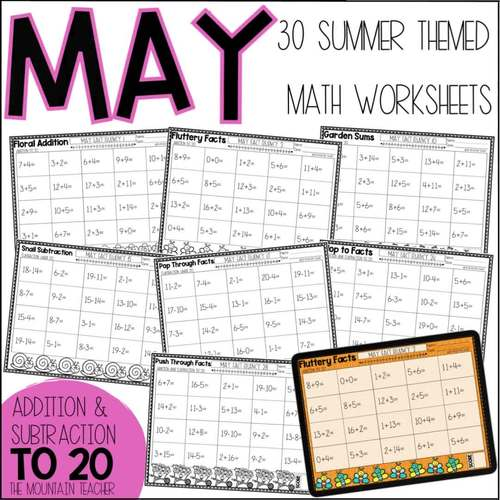# Summer Adding and Subtracting Up To 20 Worksheets | May June Math Fact Fluency

Rated 5 out of 5, based on 3 reviews
3 Ratings;
K - 3rd
Subjects
Resource Type
Standards
Formats Included
• PDF
•Google Apps™
Pages
30+
Report this resource to TPTThe Teacher-Author indicated this resource includes assets from Google Workspace (e.g. docs, slides, etc.).
Easel Activity Included
This resource includes a ready-to-use interactive activity students can complete on any device.  Easel by TPT is free to use! Learn more.
##### Also included in
1. Looking for a new DAILY routine for students to practice adding and subtracting up to 20 math fact fluency? Try these printable and digital YEAR LONG worksheets. Students will practice adding and subtracting up to 20 math fact fluency DAILY. Each month includes 30 math worksheets with 20 problems on
Price \$36.00Original Price \$40.00Save \$4.00

### Description

Looking for a new DAILY routine for students to practice adding and subtracting up to 20 math fact fluency? Try these printable and digital Summer worksheets. Students will practice adding and subtracting up to 20 math fact fluency daily throughout May or June.

This resource includes 30 math worksheets with 20 problems on each sheet:

• 10 adding up to 20 math fact fluency worksheets
• 10 subtracting up to 20 math fact fluency worksheets
• 10 adding and subtracting up to 20 math fact fluency worksheets

All worksheets include a number line to help students with their math fact fluency.

Print & Digital Options:

These adding and subtracting up to 20 math fact fluency worksheets are available in color on Google Slides for Google Classroom and are also available as black and white printable worksheets to use in person learning.

Summer Math Activities for May or June

These adding and subtracting up to 20 math fact fluency worksheets include fun summer themes including sun, pools, beaches, sunglasses, floaties, swimming, and more.

Summer adding and subtracting up to 20 worksheets have many uses:

• Daily Warm Up
• Daily Center
• Homework
• Morning Work
• Bell Work
• IEP Goals
• Ongoing Assessments
• Progress Monitoring

The PDF Includes:

• 30 Black & White Worksheets
• 30 Page Easel Activity

Looking for other daily fact fluency for a YEAR?

Looking for a different month?

This resource is designed for any elementary student working on adding and subtracting up to 20 math fact fluency.

Total Pages
30+
Included
Teaching Duration
1 month
Report this resource to TPT
Reported resources will be reviewed by our team. Report this resource to let us know if this resource violates TPT’s content guidelines.

### Standards

to see state-specific standards (only available in the US).
Add and subtract within 20, demonstrating fluency for addition and subtraction within 10. Use strategies such as counting on; making ten (e.g., 8 + 6 = 8 + 2 + 4 = 10 + 4 = 14); decomposing a number leading to a ten (e.g., 13 - 4 = 13 - 3 - 1 = 10 - 1 = 9); using the relationship between addition and subtraction (e.g., knowing that 8 + 4 = 12, one knows 12 - 8 = 4); and creating equivalent but easier or known sums (e.g., adding 6 + 7 by creating the known equivalent 6 + 6 + 1 = 12 + 1 = 13).
Fluently add and subtract within 20 using mental strategies. By end of Grade 2, know from memory all sums of two one-digit numbers.